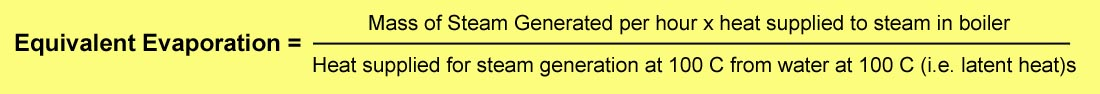# Equivalent Evaporation

Dave Wardale defined Equivalent Evaporation as follows:

Equivalent evaporation = evaporation from and at 100°C.  Evaporation figures thus expressed eliminate the effects of different feedwater and superheat temperatures, and are therefore a true measure of comparison between different boilers.  [Letter from Dave Wardale to Chris Newman, 5th April 2001.]

Equivalent Evaporation might be more simply defined as “the quantity of water at 100°C that a boiler can convert into dry/saturated steam at 100°C from each kJ of energy that is applied to it.  This defines it in terms of kg (of water/steam) per kJ of energy. However it is sometimes defined in units of kg water per kg of fuel, and (as in the case of the graph above) kg water/steam per hour.

On page 79 of his book “Red Devil and Other Tales from the Age of Steam”, Wardale includes the graph copied below which illustrates the difference between actual evaporation and equivalent evaporation (applying to an SAR Class 25 4-8-4).A more exact definition of Equivalent Evaporation in units of kg/hr comes from “Thermal Engineering” pages 608/9 by R.K. Rajput (see Google Books):

“Generally the output or evaporative capacity of the boiler is given as kg of water evaporated per hour but as different boilers generate steam at different pressures and temperatures (from feed water at different temperatures) and as such have different amounts of heat ; the number of kg of water evaporated per hour in no way provides the exact means for comparison of the performance of the boilers. Hence to compare the evaporative capacity or performance of different boilers working under different conditions it becomes imperative to provide a common base so that water be supposed to be evaporated under standard conditions. The standard conditions adopted are: Temperature of feed water 100°C and converted into dry and saturated steam at 100°C. As per these standard conditions 1 kg of water at 100°C necessitates 2257 kJ (539 kcal in MKS units) to get converted to steam at 100°C.

“Thus the equivalent evaporation may be defined as: the amount of water evaporated from water at 100°C to dry and saturated steam at 100°C.

“Consider a boiler generating ma kg of steam per hour at a pressure p and temperature T.

Let h = Enthalpy of steam per kg under the generating conditions.

• h = hf + hfg ……. Dry saturated steam at pressure p
• h = hf + xhfg ……. Wet steam with dryness fraction x at pressure p
• h = hf + hfg + cp (Tsup – Ts) ….. Superheated steam at pressure p and temperature Tsup
• hf1 = Specific enthalpy of water at a given feed temperature.

Then heat gained by the steam from the boiler per unit time = ma x (h – hf1)

The equivalent evaporation (me) from the definition is obtained as:$\frac { dS }{ d{ E }_{ b } } =\frac { { k }_{ 1 } }{ { k }_{ 2 } } -\frac { 2{ E }_{ b } }{ { k }_{ 2 } } =0$

The evaporation rate of the boiler is also sometimes given in terms of kg of steam /kg of fuel. The presently accepted standard of expressing the capacity of a boiler is in terms of the total heat added per hour.

An alternative definition is offered by Applied Thermodynamics by Onkar Singh as follows:

“For comparing the capacity of boilers working at different pressures, temperatures, different final steam conditions etc, a parameter called “equivalent evaporation” can be used. Equivalent evaporation actually indicates the amount of heat added in the boiler for steam generation. Equivalent evaporation refers to the quantity of dry saturated steam generated per unit of time from feedwater at 100°C to steam at 100°C at the saturation pressure corresponding to 100°C. Sometimes it is called equivalent evaporation from and at 100°C. Thus mathematically it could be given as:For a boiler generating steam at ‘m’ kg/h at some pressure ‘p’ and temperature ‘T’, the heat supplied for steam generation = m x (h – hw), where h is the enthalpy of final steam generated and hw is enthalpy of feedwater. Enthalpy of final steam shall be:

• h = hf + hfg = hg for final steam being dry saturated steam (hf, hfg and hg are used for their usual meanings),
• h = hf + x . hfg for wet steam as final steam,
• h = hg + cp sup.steam . (Tsup – Tsat) for superheated final steam.

Equivalent evaporation (kg/kg of fuel) =$\frac { m.(h-{ h }_{ w }) }{ 539 }$

Equivalent evaporation is thus a parameter which could be used for comparing the capacities of different boilers.”

Note – the last equation purports to express equivalent evaporation in units of kg/kg of fuel, but in fact the units are actually in kg/hr.]